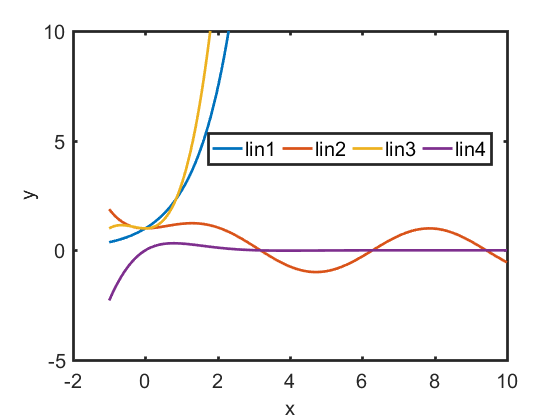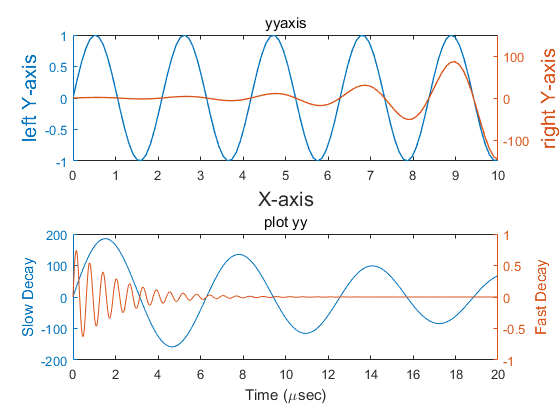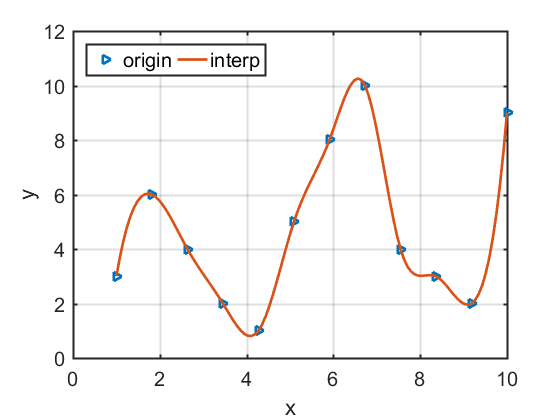create time: 2019 03 20

# Data Visualization Via MATALB 1 dimensional data

MATLAB is a power software in scientific calculations and its plot ability is also useful. I want to summarize the most frequently used MATLAB code accoring to my usage.

## plot multi lines in the same figures

The first code is how we plot multilines in the same plot. I want to add some extra settings in this code for the default settings are not so beautiful. The following is the plot of different functions:

In above figures I redefined the linewidth of the lines and ticks,changed the fontsize of legend,label etc.## Plot different lines by using different y axis

In some cases we need to plot different things in the smae plot but their number and meanings are different and are not suitable compared directly. We may need the double yy plot.## Plot the interpolation lines with its origin data

We sometimes need to draw some discrete data into a line. In order to look good, we need a smooth curve. In this case, we need to use interpolation. We then give an example to show how to plot . There are two different methods: “plotyy” and “yyaxis”